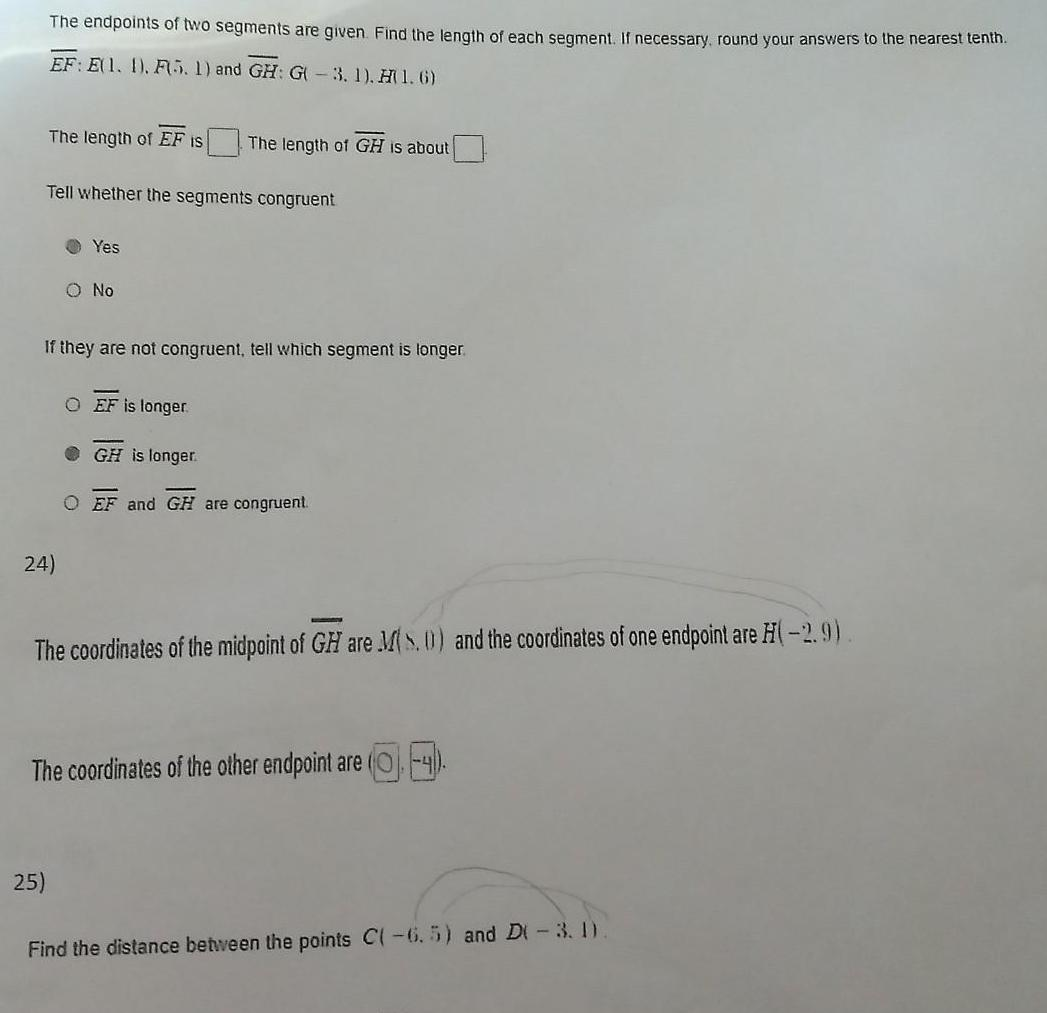Question:

# The endpoints of two segments are given Find the length of

Last updated: 9/3/2023The endpoints of two segments are given Find the length of each segment If necessary round your answers to the nearest tenth EF E 1 1 F5 1 and GH G 3 1 H 1 6 The length of EF is Tell whether the segments congruent 24 Yes 25 O No If they are not congruent tell which segment is longer The length of GH is about O EF is longer GH is longer O EF and GH are congruent The coordinates of the midpoint of GH are Ms 0 and the coordinates of one endpoint are H 2 9 The coordinates of the other endpoint are Find the distance between the points C 6 5 and D 3 1